### Home > PC > Chapter 4 > Lesson 4.1.1 > Problem4-14

4-14.
1. Rewrite each of the following equations in y = abx form. Homework Help ✎

1. y = 16(2)2x−2

2. y = (4)1/2x+2

3. y = 60 (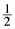)3x+1

4. y = 81 (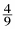)1/2x+2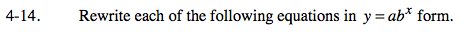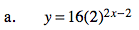$y=16\left(2^{2x}\right)\left(2^{-2}\right)$

$y=16\left(2^x\right)\left(\frac{1}{4}\right)$

$y=4\left(2^{2x}\right)$

$y=4\left(4^x\right)$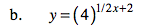See example in (a).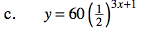See example in (a).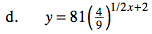See example in (a).